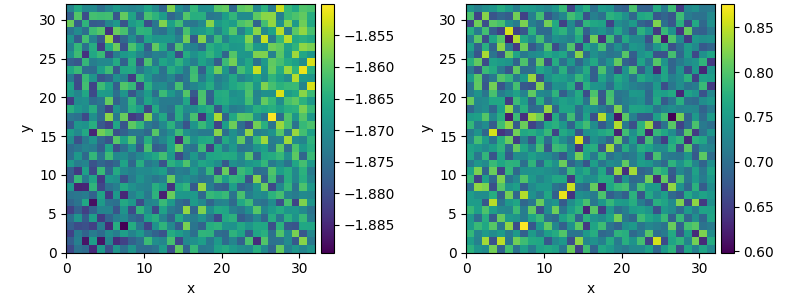# 2.20. Custom Class for coupled PDEs

This example shows how to solve a set of coupled PDEs, the spatially coupled FitzHugh–Nagumo model, which is a simple model for the excitable dynamics of coupled Neurons:

$\begin{split}\partial_t u &= \nabla^2 u + u (u - \alpha) (1 - u) + w \\ \partial_t w &= \epsilon u\end{split}$

Here, $$\alpha$$ denotes the external stimulus and $$\epsilon$$ defines the recovery time scale. We implement this as a custom PDE class below.0%|          | 0/100.0 [00:00<?, ?it/s]
Initializing:   0%|          | 0/100.0 [00:00<?, ?it/s]
0%|          | 0/100.0 [00:00<?, ?it/s]
0%|          | 0.23/100.0 [00:00<01:45,  1.05s/it]
1%|          | 0.68/100.0 [00:00<00:41,  2.38it/s]
3%|2         | 2.84/100.0 [00:00<00:16,  5.87it/s]
8%|7         | 7.7/100.0 [00:00<00:11,  8.26it/s]
15%|#4        | 14.96/100.0 [00:01<00:09,  8.89it/s]
23%|##3       | 23.34/100.0 [00:02<00:08,  9.07it/s]
32%|###2      | 32.23/100.0 [00:03<00:07,  9.51it/s]
42%|####2     | 42.06/100.0 [00:04<00:05,  9.68it/s]
52%|#####2    | 52.11/100.0 [00:05<00:04,  9.61it/s]
62%|######1   | 61.81/100.0 [00:06<00:03,  9.81it/s]
72%|#######2  | 72.12/100.0 [00:07<00:02,  9.83it/s]
82%|########2 | 82.29/100.0 [00:08<00:01,  9.81it/s]
92%|#########2| 92.17/100.0 [00:09<00:00,  9.91it/s]
92%|#########2| 92.17/100.0 [00:10<00:00,  9.14it/s]
100%|##########| 100.0/100.0 [00:10<00:00,  9.92it/s]
100%|##########| 100.0/100.0 [00:10<00:00,  9.92it/s]


from pde import FieldCollection, PDEBase, UnitGrid

class FitzhughNagumoPDE(PDEBase):
"""FitzHugh–Nagumo model with diffusive coupling"""

def __init__(self, stimulus=0.5, τ=10, a=0, b=0, bc="auto_periodic_neumann"):
super().__init__()
self.bc = bc
self.stimulus = stimulus
self.τ = τ
self.a = a
self.b = b

def evolution_rate(self, state, t=0):
v, w = state  # membrane potential and recovery variable

v_t = v.laplace(bc=self.bc) + v - v**3 / 3 - w + self.stimulus
w_t = (v + self.a - self.b * w) / self.τ

return FieldCollection([v_t, w_t])

grid = UnitGrid([32, 32])
state = FieldCollection.scalar_random_uniform(2, grid)

eq = FitzhughNagumoPDE()
result = eq.solve(state, t_range=100, dt=0.01)
result.plot()


Total running time of the script: ( 0 minutes 10.529 seconds)